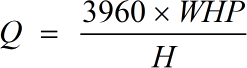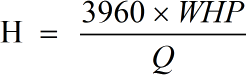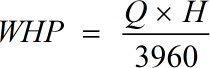Request a Tool

Water Horsepower Calculator

Water Horsepower refers to the energy or power that is added to water by the pump.

Flow Rate
0

Formula• Q = Flow Rate or Discharge
• WHP = Water Horsepower

Defination / Uses

The volume of liquid that moves in a given amount of time is measured by the flow rate of the liquid. The flow rate is determined by the area of the pipe or channel through which the liquid is travelling, as well as the liquid's velocity. The area of a liquid flowing through a pipe is A = r2, where r is the pipe's radius.

0

Formula• WHP = Water Horsepower
• Q = Flow Rate or Discharge

Defination / Uses

Pressure head and elevation head make to a fluid's total hydraulic head. The corresponding gauge pressure of a column of water at the piezometer's base is the pressure head, and the relative potential energy in terms of an elevation is the elevation head.

Water Horsepower
0

Formula• WHP = Water Horsepower
• Q = Flow Rate or Discharge

Defination / Uses

The water horsepower is the amount of power necessary to drive a centrifugal pump. It can be calculated by its flow rate and total head.

How to use brake horsepower calculator?

There are only two basic steps.

• First select the option from dropdown. What you need to calculate.
• Then add values other than requirement in their corresponding fields.

Thats it! You will get your answer in less than a single second.

How Water Horsepower Calculator is helpful

The online Water Horsepower Calculator can be used to calculate the power of water pumps in a number of different ways. This calculator can also be used to compute various water pumping factors such as HP (horsepower), flow rate or discharge, and Total Head. No sign-up, registration OR captcha is required to use this tool.